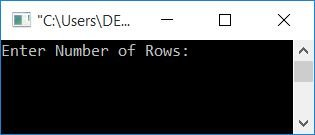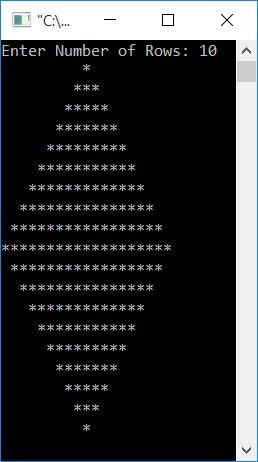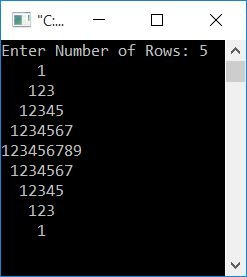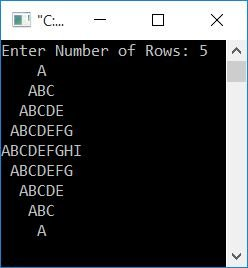# C Program to Print a Diamond Pattern

In this article, you will learn and get code about the printing of a diamond pattern in the following ways:

## Print a diamond pattern of stars

Let's create a program that asks the user to enter the row size of the upper-half diamond to print the diamond pattern of stars. For example, if the user enters 5 as the row size, then a diamond of stars of size 5*2-1 or 9 rows gets printed.

```#include<stdio.h>
#include<conio.h>
int main()
{
int i, j, row, space;
printf("Enter Number of Rows: ");
scanf("%d", &row);
space = row-1;
for(i=1; i<=row; i++)
{
for(j=1; j<=space; j++)
printf(" ");
space--;
for(j=1; j<=(2*i-1); j++)
printf("*");
printf("\n");
}
space = 1;
for(i=1; i<=(row-1); i++)
{
for(j=1; j<=space; j++)
printf(" ");
space++;
for(j=1; j<=(2*(row-i)-1); j++)
printf("*");
printf("\n");
}
getch();
return 0;
}```

This program was built and runs under the Code::Blocks IDE. Here is its output:Now enter the number of rows, say 10, to print a diamond pattern that expands up to the row-1 line. That is, with row number 10, it will print a diamond pattern with 9 lines, as shown in the output given below:#### Program Explained

If the user enters 10 as the size of the diamond, then always remember these things to print the upper half of the diamond:

• In the first row, print 9 spaces and one star.
• In the second row, print 8 spaces and three stars.
• Print seven spaces and five stars in the third row.
• Print six spaces and seven stars in the fourth row.
• Print five spaces and nine stars in the fifth row.
• Print four spaces and eleven stars in the sixth row.
• Print three spaces and thirteen stars in the seventh row.
• In the eighth row, print 2 spaces and fifteen stars.
• Print one space and seventeen stars in the ninth row.
• Print nineteen stars in the tenth row.

And for the diamond's lower half:

• Print one space and seventeen stars in the first row.
• Print two spaces and fifteen stars in the second row.
• Print three spaces and thirteen stars in the third row.
• Print four spaces and eleven stars in the fourth row.
• Print five spaces and nine stars in the fifth row.
• Print six spaces and seven stars in the sixth row.
• Print seven spaces and five stars in the seventh row.
• Print eight spaces and three stars in the eighth row.
• At the ninth row, print nine spaces and one star.

The following is the dry run of the above program: suppose user input is 10.

• The variable row is initialized with 10.
• Using the statement
`space = row-1;`
9 gets initialized to "space."
• The for loop is now being executed.
• At the first run of the for loop with i = 1, the dry run goes like this:
1. Inside the loop, 1 gets initialized to i and checks whether it is less than or equal to row or not
2. Inside the loop, 1 gets initialized to i, and it is checked whether it is less than or equal to "row" or not.
3. Because the value of i (1) is obviously less than the value of row (10), the condition evaluates to true.
4. Therefore, program flow goes inside the loop's body and executes another for loop.
5. This time, 1 gets initialized to j and checks whether it is less than or equal to the value of space (9) or not.
6. The condition is satisfied.
7. Therefore, program flow goes inside the loop body and executes only one statement, that is,
`printf(" ");`
that prints a space.
8. Now the variable j gets incremented, and again, program flow goes to the loop's condition part and checks whether the updated value or incremented value of j (2) is less than or equal to the value of space (9) or not.
9. The condition is again evaluated as true. Therefore, program flow again goes inside the loop and prints another space.
10. In this way, the printing of space continues until the value of j becomes greater than the value of space.
11. As a result, the first row contains a total of 9 spaces.
12. And then decrement the value of space to print one less space on the next row than the previous one.
13. Now the program flow goes to the second for loop part (present inside the first outer for loop).
14. There, 1 gets initialized to j and checks whether it is less than or equal to the value of ((2*i)-1) or not.
15. Because the value of i was 1 in the first run, compare j to (2*i)-1, (2*1)-1, or 1.
16. The condition is satisfied.
17. Therefore, program flow goes inside the loop and prints a star (*).
18. Then it increases the value of j and compares it to ((2*i)-1).
19. This time, the value of j (2) is not less than or equal to (2*i)-1, which is 1.
20. Therefore, the condition evaluates to false, and the program flow exits the loop.
21. The program flow now begins the next output (printing) thing from the new line by using the newline character ('\n').
• At the second run of the for loop with i = 2, the dry run goes like this:
• Print 8 spaces using first for loop.The process goes the same from step no. 1 to step no. 10 as mentioned above, with an updated value of i.
• Again, decreasing the value of space to print one less space next time. Now space=8.
• This prints three stars by using second for loop.The process continues from step 12 to step 19 as described above, but with an updated value of i.
• Then proceed to step 20.
• At the third run of the for loop with i=3, the dry run looks like this:
• Process the similar operation from step no. 1 to step no. 10 with the new value of i (3). That is, 7 spaces are printed.
• Reduces the value of space. Now space=7.
• Process the similar operation given from step no. 12 to step no. 19 with the new value of i (3). That is, print 5 stars.
• Process step no. 20
• Continue executing the loop until the value of i becomes 11.
• Because 11 is not less than or equal to row 10, the condition evaluates to false, and the program flow exits from this loop.
• Print the lower half of a diamond in the same manner with the initial value of space as 1.
• Because we only need to print one space in the first row of the lower half.

## Print a diamond pattern of numbers

Here is another program for printing diamond-shaped numbers. In each row, the number starts with 1. The program is similar to the previous one. Except, in place of the star, print the number using a variable named "num" (initialized with 1 at the start of the program). Increment the value of num after each print.

Remember to always initialize num with 1 after the statement `printf("\n");` to begin with 1 for each row.

```#include<stdio.h>
#include<conio.h>
int main()
{
int i, j, row, space, num=1;
printf("Enter Number of Rows: ");
scanf("%d", &row);
space = row-1;
for(i=1; i<=row; i++)
{
for(j=1; j<=(space); j++)
printf(" ");
space--;
for(j=1; j<=(2*i-1); j++)
{
printf("%d", num);
num++;
}
printf("\n");
num=1;
}
space = 1;
for(i=1; i<=(row-1); i++)
{
for(j=1; j<=(space); j++)
printf(" ");
space++;
for(j=1; j<=(2*(row-i)-1); j++)
{
printf("%d", num);
num++;
}
printf("\n");
num=1;
}
getch();
return 0;
}```

Here is the output produced by the above program, assuming that the user has entered 5 as the size of the diamond:## Print a Diamond Pattern of Alphabets

This program is nearly identical to the previous one. In place of a number, use a character using a variable, say, ch of the char type. Initialize it with A. The rest of the program is similar to the previous one.

```#include<stdio.h>
#include<conio.h>
int main()
{
int i, j, row, space;
char ch='A';
printf("Enter Number of Rows: ");
scanf("%d", &row);
space = row-1;
for(i=1; i<=row; i++)
{
for(j=1; j<=(space); j++)
printf(" ");
space--;
for(j=1; j<=(2*i-1); j++)
{
printf("%c", ch);
ch++;
}
ch='A';
printf("\n");
}
space = 1;
for(i=1; i<=(row-1); i++)
{
for(j=1; j<=(space); j++)
printf(" ");
space++;
for(j=1; j<=(2*(row-i)-1); j++)
{
printf("%c", ch);
ch++;
}
ch='A';
printf("\n");
}
getch();
return 0;
}```

Here is its output, assuming the user input is 5:#### The same program in different languages

C Quiz

« Previous Program Next Program »